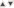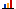Qur’an Tools is not optimised for narrow screens

The Formulaic Density and Usage Statistics per Sura report, found under the “Formulae” menu lists each of the 114 suras in the Qur’an along with information related to the formulaic phrases found in that sura. It looks like this:1 Formula Length and Type. You can choose the type of formulae that Qur’an Tools counts and shows in the report. (The length is the number of Arabic words in a formula; the type allows you to choose from any of the three formula types that Qur’an Tools understands). Learn more about formula lengths and types here. 2 Sura Number and Provenance. Each sura has its number and provenance (Meccan or Medinan, according to the commonly used Nöldeke-Schwally-Robinson system) listed. Click on the sura number to see verse by verse detail of the formulae in the sura. 3 Roots. The number of Arabic roots in each sura. (You can sort by this, and indeed any column, by using thebuttons at the top of each column). 4 Roots Part of a Formulae. Shows the number of Arabic roots in this sura that are part of a formulaic phrase. (This is used, along with the total number of roots (see #3 above) to calculate the formulaic density of this sura, which is defined as [Number of Roots Part of a Formulae] / [Total Number of Roots]. TIP Click on any number in this column to see a list of all the formulae in the sura. 5 Number of Formulae Used. Reports the total number of formulae used in this sura, including duplicates (so, for example, if a sura used just one formulae 10 times, this column would show "10". You can see a chart of this column by clicking theicon at the top of the column. 6 Diversity of Formulae Used. Reports the total number of different formulae used in this sura, including duplicates (so, for example, if a sura used just one formulae 10 times, this column would show "1". You can see a chart of this column by clicking theicon at the top of the column. 7 Formulae Unique to Sura. Counts how many formulae only occur in this sura. You can see a chart of this column by clicking theicon at the top of the column. 8 Formulaic Density. The formulaic density of this sura (the percentage of words in it that are part of a formulae). You can see a chart of this information by clicking theicon at the top of the column (or theicon on an individual row, to see a chart of the formulaic densities of each verse in that sura).